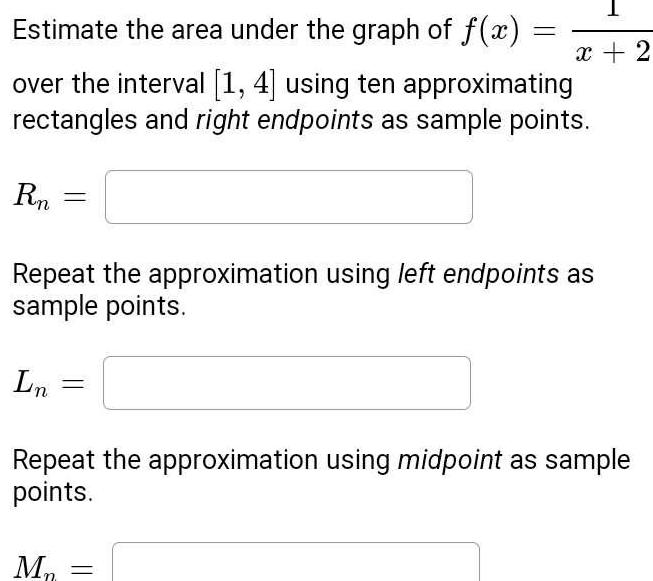Question:

# Estimate the area under the graph of f x over the interval 1

Last updated: 11/19/2023Estimate the area under the graph of f x over the interval 1 4 using ten approximating rectangles and right endpoints as sample points Rn Ln Repeat the approximation using left endpoints as sample points x 2 Repeat the approximation using midpoint as sample points M